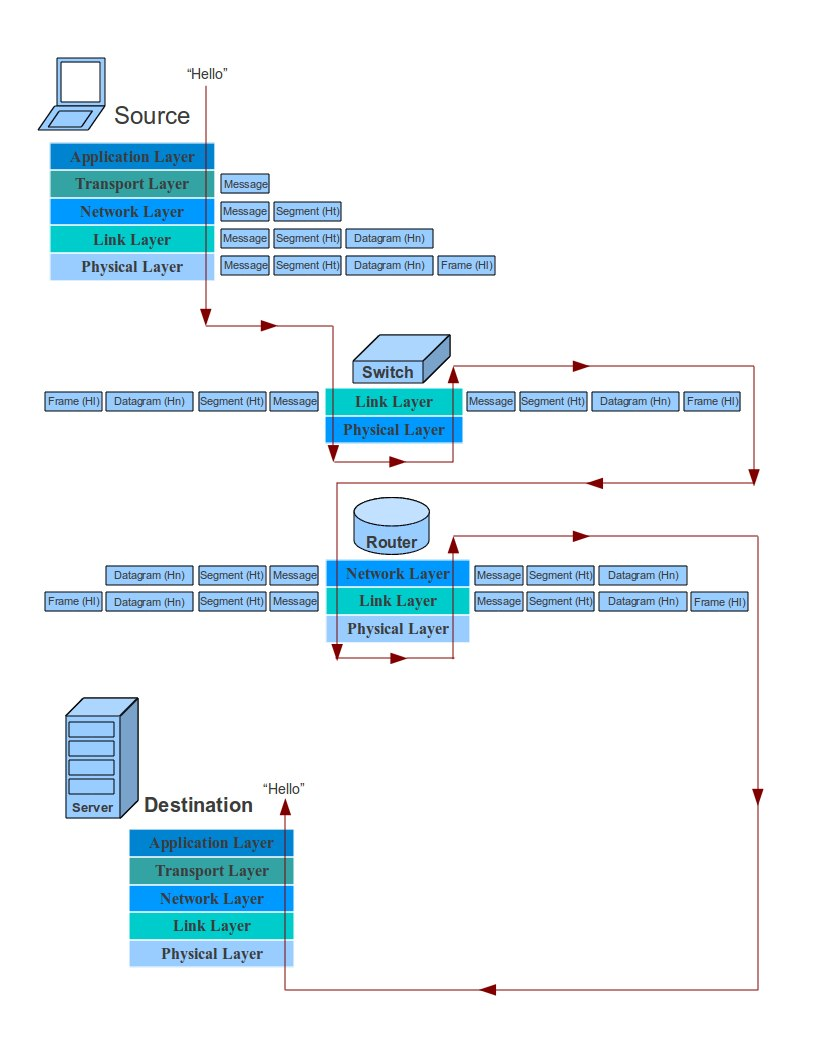Introduction to Network Security – Part 6

NOTIFICATION: These examples are provided for educational purposes. The use of this code and/or information is under your own responsibility and risk. The information and/or code is given ‘as is’. I do not take responsibilities of how they are used.

Poly-alphabetic Cipher

In the previous posting, we say that the mono-alphabetic cipher instead of shifting the alphabet a number of letters (Caesar cipher), its substitute each letter arbitrarily by mapping the plaintext letter map to a random arranged ciphertext. The only requirement for the ciphertext was that the letters must not be repeated. Now we are going to see a cipher that uses a set of related mono-alphabetic rules plus a key to determine which rule will be use to perform a transformation.

Vigenère Cipher

Encryption:

This cipher is similar to the Caesar cipher for the use of the 26 letters alphabet with the only different that we create a table in which:

1. The columns represent the plain text
2. The rows represent the key
3. The alphabet inside the table is shifted to the right one letter one time for each letter of the alphabet key.

To be more clear, let take a quick look of the Caesar cipher table: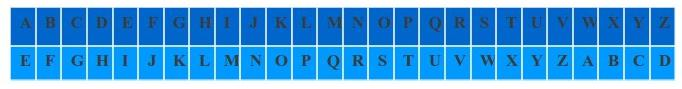In this example, We started the alphabet on the letter ‘E’ because the key was 5.

Now, the Vigenère Cipher will apply this shifting 26 times, one time per row, for each letter of the alphabet that correspond to the key as follow: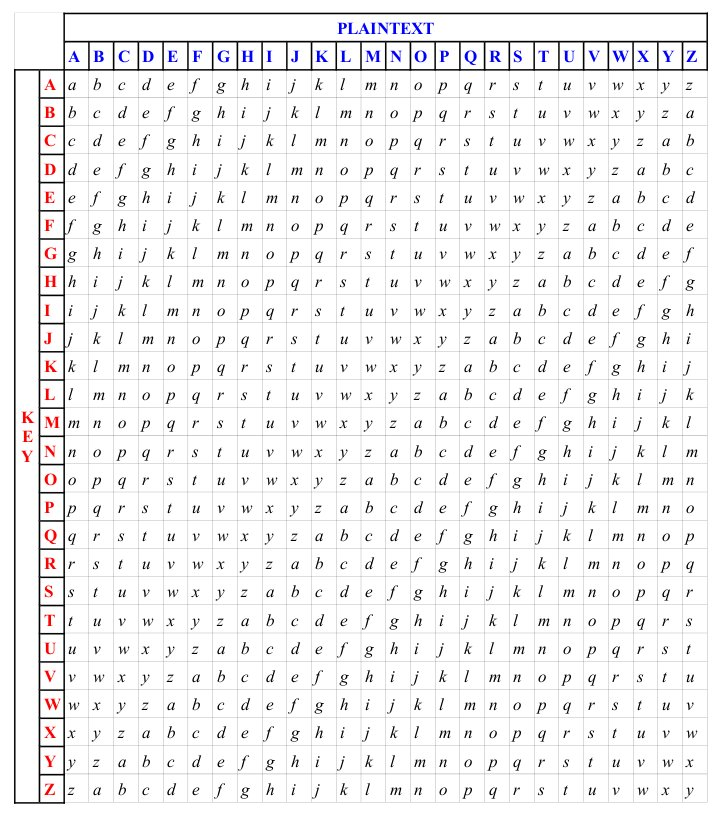Lets say that you have the following key “THIS  MESSAGE WAS FOR YOU”, and your key is “HELLO” then using the table: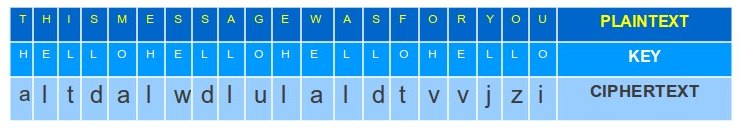From a mathematical point of view we have:

1. Lets assume that we take the letters of the alphabet from A to Z and be replace them with number starting from 0, for example: A = 0, B = 1, …, Z = 25.
2. Since we have 26 letters in the alphabet, lets perform module of 26 on this equation.
3. If ‘i’ is the letter position, P indicate the plaintext, K indicate the key, and C indicate the ciphertext then:Decryption

For decryption we only need to use a letter of the key to identify the row and the letter of the ciphertext in the row to identify the column, the letter designated to the column give us the plaintext letter.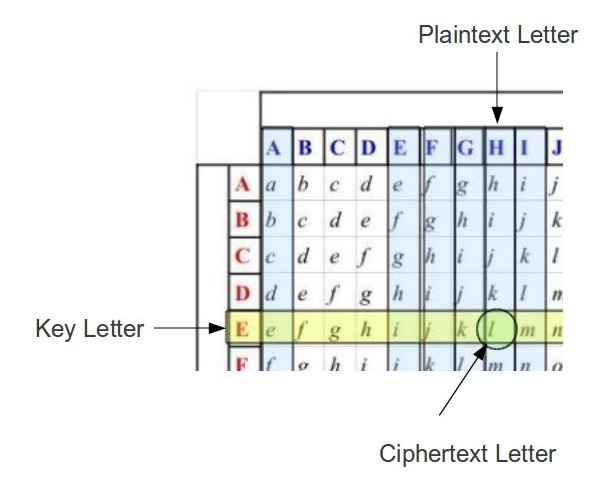From a mathematical point of view we have:

1. Lets assume that we take the letters of the alphabet from A to Z and be replace them with number starting from 0, for example: A = 0, B = 1, …, Z = 25.
2. Since we have 26 letters in the alphabet, lets perform module of 26 on this equation.
3. If ‘i’ is the letter position, P indicate the plaintext, K indicate the key, and C indicate the ciphertext then:Security

This cipher is not secure. If two or more sequences are identical inside the plaintext, we run the risk that identical ciphertext sequence will be generated. The attacker can use these repetition in the ciphertext to make a deduction about what is the plaintext. The more plaintext is needed to encrypt, the more chances that the ciphertext can be broken or the key found.

As an example, lets assume we have the following:
Plaintext:   WE RUN WHEN WE WERE DISCOVER BY THEM
Key:             RUNNING NO RUNNING NO RUNNING NO

This would give us a ciphertext in which we can spot the repetitions: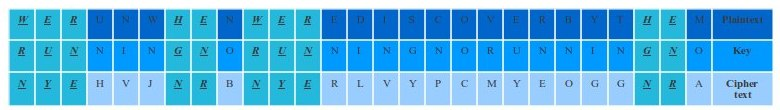The only way around this problem is by using the Autokey cipher.

Autokey Cipher

An auto-key cipher is the concept of generating a key that does not have a repetition cycle.

Instead of having a plaintext and a key such as this example:
Plaintext:   WE RUN WHEN WE WERE DISCOVER BY THEM
Key:             RUNNING NO RUNNING NO RUNNING NO

We could have the following key:
Plaintext:   WE RUN WHEN WE WERE DISCOVER BY THEM
Key:             RUNNING IS NOT THE SOLUTION THIS

This would give us a ciphertext with no repetitions:

The One-Time Pad cipher use a similar concept as the Auto-Key Cipher; however, the difference is the generation of a random key which is as long as the message. Also, it is required that at the end of the transmission, the random key generated must be destroyed.

The only problem is to find a secure way to distribute the random generated key between the principals.

Introduction to Network Security – Part 5

NOTIFICATION: These examples are provided for educational purposes. The use of this code and/or information is under your own responsibility and risk. The information and/or code is given ‘as is’. I do not take responsibilities of how they are used.

Symmetric Encryption

In the symmetric encryption, the same key (normally a single-key) is used to perform the encryption and decryption of the ciphertext.

Symmetric Cipher Model: This model is performed by performing transformations and substitutions on the plaintext. A secret key, independent from the plaintext and the algorithm, is used to cipher the plaintext. After, the ciphertext plus the secret key is used with the decryption algorithm to obtain the original plaintext.

Symmetric Encryption is the opposite to the concept of public key distribution which will be explained in future postings.

Requirements:

1. The cipher model must be mathematical expression:
(E: Encryption, D: Decryption, X: plaintext, Y: ciphertext, K: secret key)

Y = E(K, X)
X = D(K, Y)

2. Assumption that the encryption algorithm is known to the attacker.
3. A strong encryption algorithm which in case the attacker would obtain or know some examples of the ciphertext and the plaintext produced from the ciphertext, the attacker would still be not able to obtain the key. This means that if the attacker would obtain the ciphertext, the attacker would not be able to obtain the secret key or the plain text.
4. Secret key should be known only by the sender and the receiver of the ciphertext.
5. The distribution of the secret key must be done in a secure fashion. For example, the use of a third party that would generate and provide in a secure way the key to the sender and the receiver.

Substitution Ciphers

In classical substitution ciphers, all the letters in the plaintext will be replaced by another letter, number, and/or symbol.

Caesar Cipher

History explains that Julius Caesar <http://www.roman-empire.net/republic/caesar-index.html> came up with a substitution cipher that he used in his campaigns for military affairs.

The cipher works in the following way:

1. We use the alphabet of 26 letters: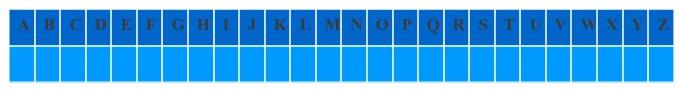2. Under this alphabet, we will rewrite the alphabet by picking a letter as a starting point.
Lets say our key indicate the starting point such as K = 4 so we begin with the letter ‘E’ then:3. This means that if we wish to send a plaintext (P) that says HELLO, the ciphertext (C) would be LIPPS, and the key (K) would be 4
4. The mathematical way to represent this cipher will be the follows:
1. Give each letter of the alphabet a number:
A = 1, B = 2, C = 3, D = 4, E = 5,F = 6, G = 7, H = 8, J = 9, K = 10, L = 11, M = 12, N = 13, O = 14,P = 15, Q = 16, R = 17, S = 18, T = 19, U = 20, V = 21, W = 22, X = 23, Y = 24, Z = 25.
2. Encryption Algorithm:
E: Encryption, Ct: Ciphertext, Pt: Plaintext, K: secret key

Ct = E(Pt)
= (Pt + K) mod 26
3. Decryption Algorithm:
D: Decryptor, Ct: Ciphertext, Pt: Plaintext, K: secret key

Pt = D(Ct)
= (26 + (Ct - K)) mod 26
5. The weakness of this cipher is that it can be broken by brute force. We just need to test the 25 combinations of different keys  until we find the key that reveals the message.

Monoalphabetic Cipher

The mono-alphabetic cipher instead of shifting the alphabet a number of letters, its substitute each letter arbitrarily by mapping the plaintext letter map to a random arranged ciphertext. The only requirement for the ciphertext is that the letters must not be repeated.

Since we are using 26 letters of the alphabet the arrangement of the cipher can permute a total of 26! permutations.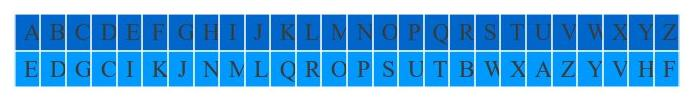If we wish to encode the word “HELLO”, we would obtain “NERRS”

Lets assume we wish to cipher a plaintext:

Plaintext = “THIS IS A SECRET MESSAGE ENCODED IN MONOALPHABETIC”

Ciphertext = “XNMW MW E WIGBIX OIWWEJI IPGSCIC MP OSPSERUEDIXMG”

The following website let you play a little with monoalphabetic cipher by randomizing for you the ciphertext:

The only problem is that this cipher can be exploited by doing regularities analysis over the frequency of the letters. Base on the language rules some letters are used more than others. For example, in English, the letter ‘E’ is the most common used in words, followed by A, I, O, N, R, S, T. Others letters such as K, J, Q, X, Z are less used than the rest.

The largest is the message, the most chances that the attacker can decrypt the message.
Just in this message “XNMW MW E WIGBIX OIWWEJI IPGSCIC MP OSPSERUEDIXMG” we have:

• W = 6 letters
• E = 4 letters
• M = 4 letters
• S = 3 letters
• P = 2 letters
• ….

And continue counting.

As you may notice the letter ‘W’ of the encrypted message have the most counts, so we could  assume that this is the letter E of the plaintext.

If you are interested to know the frequency of letters in English you can go to the following website:
<http://www.cryptograms.org/letter-frequencies.php>

<http://unsecure.co.uk/attackingmonoalphabeticciphers.asp>

Playfair Cipher

Playfair is one way to improve the security of mono-alphabetic cipher by encrypting multiple letters.

Playfair Encryption

1. Create a playfair key matrix:
1. Create a matrix of letters based on a keyword. For this example, the matrix should be 5 by 5
2. Fill in the letters of the keyword from left to right and from top to bottom. Make sure that there are not duplicate letters
3. Fill the rest of the matrix with the other letters that are not in the keyboard, making sure to not duplicate letters.
4. As a rule, the letter I and J count as one letter.
• I am not sure the reason for this rule, except the following:
• First, it make it harder to decrypt the message since one letter is missing.
• Second, in some languages, the J and I would have the same pronunciation.
For example, my last name Carlstein was originally written as Karlštejn.
• In case you know the real reason, please let me know and give me a reference to verify (thanks).
5. Example of playfair key matrix:
1. Let use the keyword: “EDUCATOR”
2. The table should looks like this: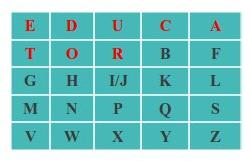3. Notice that I and J are counted as one letter
2. The next step is to encrypt the plaintext taking two letters at the time.
1. In case a two letters are the same (repeated), we must insert a filler letter (use the letter X as the filler). For example:
HELLO → HE LX LO
2. In case two letters are in the same row, replace each letter with the letter to the right. In case the letter is at the last column, pick the letter of the first row (the table is considerate to be circular). For example, lets say we have the letters D and A:1. D → U and A → E
2. Therefore DA became UE
3. In case two letters are in the same column, replace each letter with the letter below. In case the letter is at the last row, pick the letter of the first row (the table is considerate to be circular). For example, lets say we have the letters T and V:1. T → G and V → E
2. Therefore TV became GE
4. In case two letter are in different row and column, the first letter will be replaced with another letter of the same row on the column of the second letter. The second letter will be replaced with another letter of the same row on the column of the first letter. For example lets say we have the letters O and Q: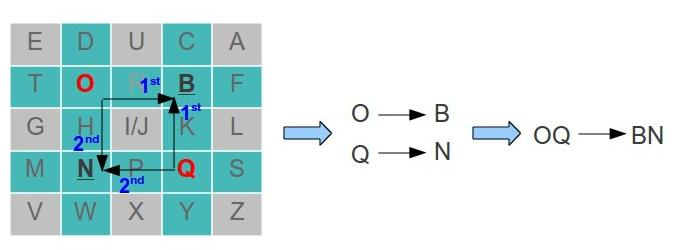1. To replace the letter O:
1. This means that O → B
2. To replace the letter Q:
1. This means that Q → N
3. Therefore OQ became BN

Playfair Decryption:

1. Decrypt two letters at a time:
1. In case two letters are in the same row, replace each letter with the letter to the left. In case the letter is at the last column, pick the letter of the first row (the table is considerate to be circular). For example, lets say we have the letters U and E: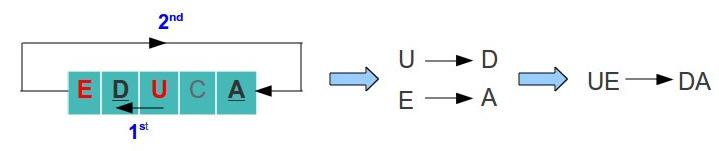1. U → D and E → A
2. Therefore UE became DA
2. In case two letters are in the same column, replace each letter with the letter above. In case the letter is at the last row, pick the letter of the first row (the table is considerate to be circular). For example, lets say we have the letters G and E: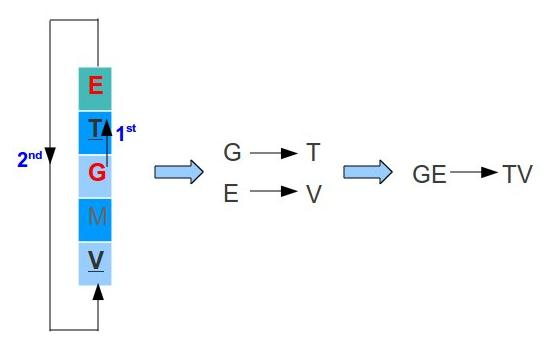1. G → T and E → V
2. Therefore GE became TV
3. In case two letter are in different row and column, the first letter will be replaced with another letter of the same row on the column of the second letter. The second letter will be replaced with another letter of the same row on the column of the first letter. For example lets say we have the letters B and N: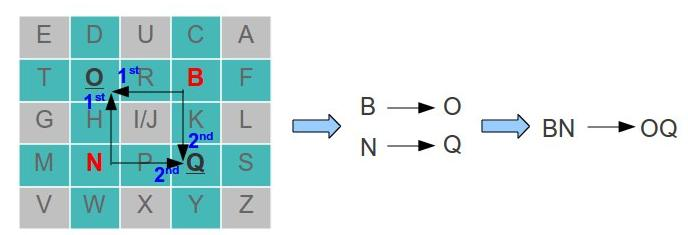1. To replace the letter B:
1. This means that O → B
2. To replace the letter Q:
1. This means that Q → N
3. Therefore OQ became BN
2. After you will finish with the final message. You must remove any extra X that do not make sense in the message:
HE LX LO → HELLO

Introduction to Network Security – Part 4

NOTIFICATION: These examples are provided for educational purposes. The use of this code and/or information is under your own responsibility and risk. The information and/or code is given ‘as is’. I do not take responsibilities of how they are used.

Before we begin talking about encryption, decryption, and ciphers related topic, let go over some terminologies to have in account:

• Cipher: An algorithm used for encryption.
• Ciphertext: The encrypted(coded) message.
• Cryptanalysis: Study of the principles and methods of deciphering a ciphertext without having the required key.
• Cryptography: Study of the principles and methods of encryption.
• Cryptology: The study of cryptanalysis and cryptography.
• Deciphering: Also known as decryption. The act of transforming a ciphertext to the original plaintext.
• Decryption: Also known as deciphering. The act of transforming a ciphertext to the original plaintext.
• Enciphering: Also known as encryption. The act of transforming a plaintext to a ciphertext.
• Encryption: Also know as enciphering. The act of transforming a plaintext to a ciphertext.
• Plaintext: the original message to be encrypted.
• Product: stages of transposition and substitutions performed.
• Secret key: An input required for the encryption and/or decryption algorithms.
• Substitution: Map each element in a plain text to another element.
• Transposition: Rearrange the elements in the plaintext

Cryptography

A cryptographic system is characterized by the use of encryption operations, number of keys used for encryption and decryption, and the way in which the plain text is processed.

Encryption Operations: In order to encrypt a plaintext to a chipertext is required to perform multiple stages of transposition and substitution, also known as product.

• Substitution: We take each element from the plaintext and mapped them to another element
• Transposition: We  take each element in the plaintext and rearrange its order in such a way that it differ from the original plaintext.

To perform encryption and decryption, we use a key reference. We can categorize the encryption techniques as  symmetric, single, asymmetric, double, and/or public.

The plaintext can be processed by using a method of streams or blocks:

• Stream: The plaintext is processed as a continuous set of elements in which each element is encrypted one at a time.
• Blocks: The plaintext is divided in a set of blocks in which each block is encrypted one at a time.

Cryptanalysis

As explained in the terminology list, Cryptanalysis is purpose of decrypt an encrypted ciphertext without the knowledge of the key used for the encryption. One way is to attack the encryption system and recover the key used for the encryption instead of recovering the plaintext from a single ciphertext.
Cryptanalysis attacks are divided in two categories:

1. Brute-force Attack: Every combination of a possible key is tested on the chipertext until the plaintext is obtained.
2. Cryptanalytic Attack: The use of knowing some characteristic of the original plaintext such as some used keywords, language, format, plaintext to ciphertext pairs examples, and  knowledge of the possible algorithm used to decrypt the ciphertext.

Unconditional Security

We call unconditional security when a cipher cannot be broken by using a ciphertext and the plaintext that produced the ciphertext regardless of the computational power and time available. Up to day, there are no encryption algorithm that can be unconditional secure with the exception of the one-time pad encryption algorithm <http://www.ibm.com/developerworks/library/s-pads.html> which will be explained in the following postings.

Computational Security

Base on the cost-benefit of braking a cipher, a cipher may not be broker due:

1. The cost of braking the cipher is greater than the value of the plaintext encrypted
2. The time required to breaking the cipher exceed the usefulness lifetime of the plaintext encrypted
3. Depending of the complexity of the cipher, there would be a limitation of computing resources and time.

Brute Force Search

As explained before, we call brute force to try every key combination possible to decrypt the ciphertext into plaintext. Before obtaining success, the attacker must try at least 50 percent of the possible keys; therefore, the probability of success may be proportional to the size of the key.

Lets assume we wish to have to option of using:

2. Triple DES (168-bit) <http://en.wikipedia.org/wiki/Triple_DES>
3. AES (Greater than 128 bits) <http://www.aescrypt.com/>

Depending of which encryption we use, the time required to find the right key by brute force could be: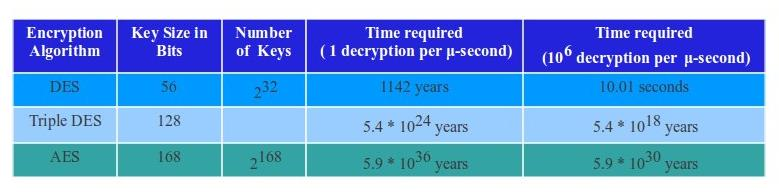Introduction to Network Security – Part 3

NOTIFICATION: These examples are provided for educational purposes. The use of this code and/or information is under your own responsibility and risk. The information and/or code is given ‘as is’. I do not take responsibilities of how they are used.

Socket Programming

In networks applications, communications are almost always done using a client-server model.

A client will perform request for a service to the server, and receive a service from the server (which should be usually on). The only thing that the client needs to know is the address of the server and which port to use. Once the connection between the client and the server is establish, then the client and the server can send and receive information back and forwards. Eg. FTP client to a FTP server

The client usually should communicate with only one server at a time; however, there are exceptions such as the web browser. In today web browsers, a web browsers could connect to different servers in order to download all the elements of a page faster. A page could indicate that the images are from one server, while the flash animation is coming from a different server.

In order to a client and a server to communicate they must use sockets in order to send and receive messages from each other. A socket is used by a sending process to send a message to the transport infrastructure which deliver the message to a receiving process which also is using a socket to receive the message.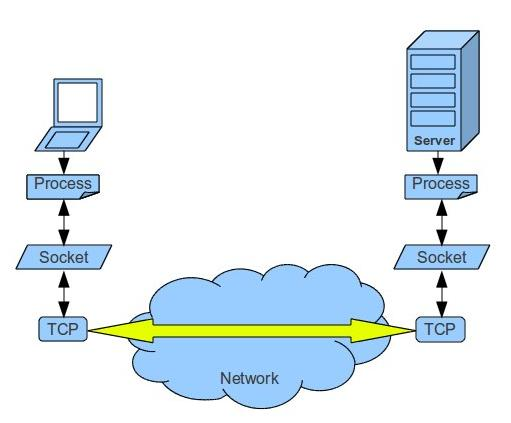The first step for a process that is receiving a message is to have an identifier. This identifier must include the IP address and a port number. The port number must be associated with the process. The port number is a 16-bit number used to identify the application process. Notice that an IP address do not identify a process but the port does, so you can have more than one process running at the host which means different ports can be used at the same time with the same IP address.

Right now, October 27 of 2010, there are two Internet Protocols (IP) available: IPv4 and IPv6.

1. IPv4 (IP version 4) is a 32-bit binary number represented by using 4 decimal values, separated by periods, in dotted decimal notation. Each decimal value is an octed (8-bits) which range between 0 to 255. Example: 192.168.1.101  would be 11000000.10101000.00000001.01100101.
1. 192 → 11000000
2. 168 → 10101000
3. 1      → 00000001
4. 101 → 01100101
2. IPv6 (IP version 6) is designed to succeed IPv4 (IP version 4). Instead of a 32-bit binary number, IPv6 use 128-bits. This means that the use of Notation Address Translation (NAT) is not needed as in IPv4. Network security such as IPSec (http://en.wikipedia.org/wiki/IPsec) which allow to authenticate and encrypt IP packages have being incorporated.

Ports

As explained previously a port is a 16-bit identifier number which identify the type of application process, this means that there are a total number of 65535 ports number available. For more information you can go to the Internet Assigned Numbers Authority (IANA) <http://www.iana.org> . This institution is in charge of the global coordination of the DNS Root, IP addressing, and other Internet protocol resources.

1. Ports from 0 to 1023 are called well-known ports.
1. Examples of reserved ports: HTTP (Port 80), FTP (Port 21), Telnet (Port 23), STMP (Port 25), etc.
2. Ports from 1024 through 49151 are called reserved ports.
3. Ports from 49152 to 65535 are called Dynamic ports, Private ports, or Ephemeral ports.

TCP Socket on Client Side.

1. Create a socket to create an endpoint for communication.
1. (In Linux) ANSI C:
int socket(int domain, int type, int protocol);
2. Specify the server’s IP address and port to connect
3. Establish a connection initiate a connection on a socket with the server.
1. (In Linux) ANSI C:
4. Send data to the server and receive data from the server
5. Close the connection
Here is an example of a client/server program using ANSI C in Linux: <http://www.acarlstein.com/?p=891>
TCP Socket on Server Side
1. Create a socket to create an endpoint for communication.
1. (In Linux) ANSI C:
int socket(int domain, int type, int protocol);
2. Bind the socket with an address to specify the server’s IP address and port.
1. (In Linux) ANSI C:
3. Listen form incoming connections.
1. (In Linux) ANSI C:
int listen(int sockfd, int backlog);
4. Accept the connection with the client.
1. (In Linux) ANSI C:
5. Send data to the server and receive data from the server
6. Close the connection

Here is an example of a client/server program using ANSI C in Linux: <http://www.acarlstein.com/?p=891>

Introduction to Network Security – Part 2

NOTIFICATION: These examples are provided for educational purposes. The use of this code and/or information is under your own responsibility and risk. The information and/or code is given ‘as is’. I do not take responsibilities of how they are used.

Introduction To Network : The Internet

The Internet is a network which connect networks around the world. Each end system (normally end users computers) obtain access thought an Internet Service Provider (ISPs).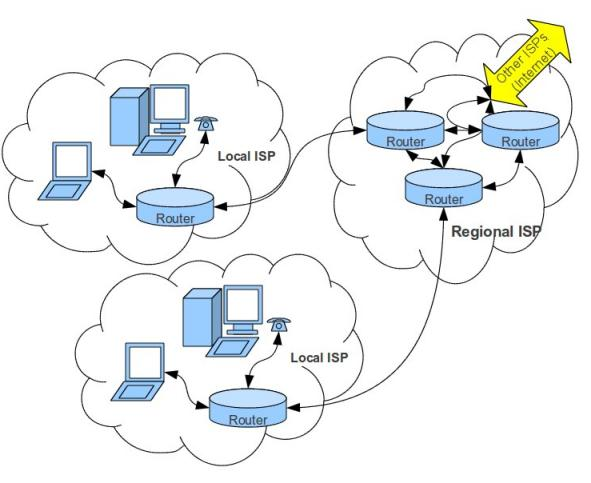Protocols

A protocol is a way in which rules for communication are established. For example, in a court, there is a procedure and language that must be follow in the process. A network protocol will define a language of rules, format, order of messages, entities, actions to take, and convention in order to the message being transmitted.

For example: A user which to see a website, therefore the browser will make a TCP request to the server. Due the TCP request form the client, the server answer with a TCP response to the client. Then the client perform a request for the page to the server. Finally, the server send the page requested by the client.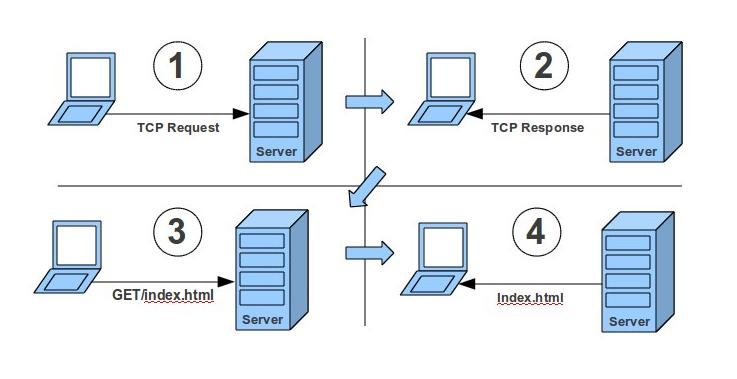Protocol Layers

A protocol divided the components needed for transition of messages in modular layers (modulation of the components helps to maintain, update, and/or perform changes to the component). The implementation of layers must be done in the way that it is transparent to the rest of the system as will be explained later. The following image provide an example of the actual layers used for the TCP/IP protocol and the protocol layers recommended by the OSI model.As you can witness, in the actual TCP/IP protocol, we have five well defined layers.

This layers are:

1. Application Layer: This layers permit the user to access the information that is being send and/or receive from the network through the application in used for this purpose.
1. This layer support application-layer protocols such as Hyper-Text Transfer Protocol (HTTP), File Transfer Protocol (FTP), Secure  SHell (SSH), and others.
2. The information to be transmitted is send over the application layers which is in charge to encapsulate the data into the application layer protocol and transfer the encapsulated data to the transport layer.
2. Transport Layer: This layer is in charge to provide to end users a transparent transfer of data.
1. This layer provide controls of reliability of a given link through flow control segmentation/de-segmentation plus error control.
2. The most common segments used today are Transmission Control Protocol (TCP) and User Datagram Protocol.
1. Transmission Control Protocol (TCP) is know to be a reliable connector-oriented protocol. Commonly used to send data.
2. User Datagram Protocol  (UDP) is know to be a faster but unreliable connector-oriented protocol. Commonly used for streaming media such as radio, movies, clips, and TV online.
3. Network Layer: This layer is in charge of routing the datagrams from the source to the destination using routing protocols such as Internet Protocol (IP). All routes operate at this layer.
4. Link Layer: This layer is used for the transfer of data between network entities such as bridges and switches by providing the functional and procedural means.
5. Physical Layer: All the data is encoded and transmit as raw data over the network media by making sure that the information that is send in one side is received at the other side accurately.